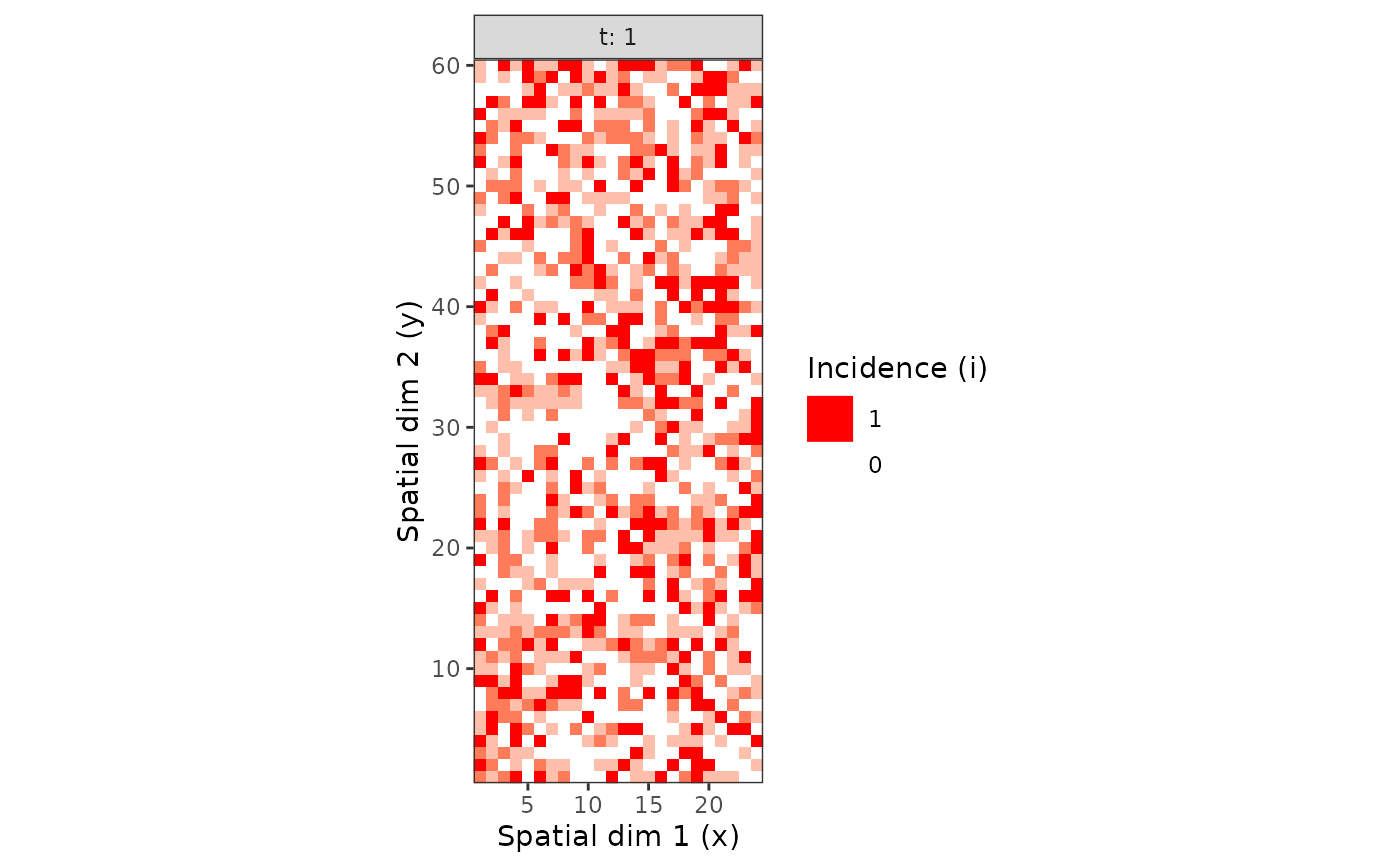This function provides a easy way to regroup recorded data into groups of same number of individuals.

clump(object, ...)

# S3 method for intensity
clump(object, unit_size, fun = sum,
inclusive_unspecified = FALSE, ...)

## Arguments

object An intensity object. Additional arguments to be passed to fun. Size of a group unit. It must be a named vector, with names corresponding to non-observational variables (i.e. space and time variables). If the size of a variable in the data set is not a multiple of the provided value in unit_size, some sampling units (the last ones) will be dropped so that clumps of individuals remain even throughout the data set. Function used to group observational data together. Not yet implemented. Do unspecified mapped variables (different from i and n) need to be included into the bigger possible sampling unit (TRUE) or splited into as many sampling units as possible (FALSE, default)?

## Examples

my_incidence <- incidence(tomato_tswv\$field_1929)
plot(my_incidence, type = "all")#> Warning: Computation failed in stat_summary():
#> Hmisc package required for this function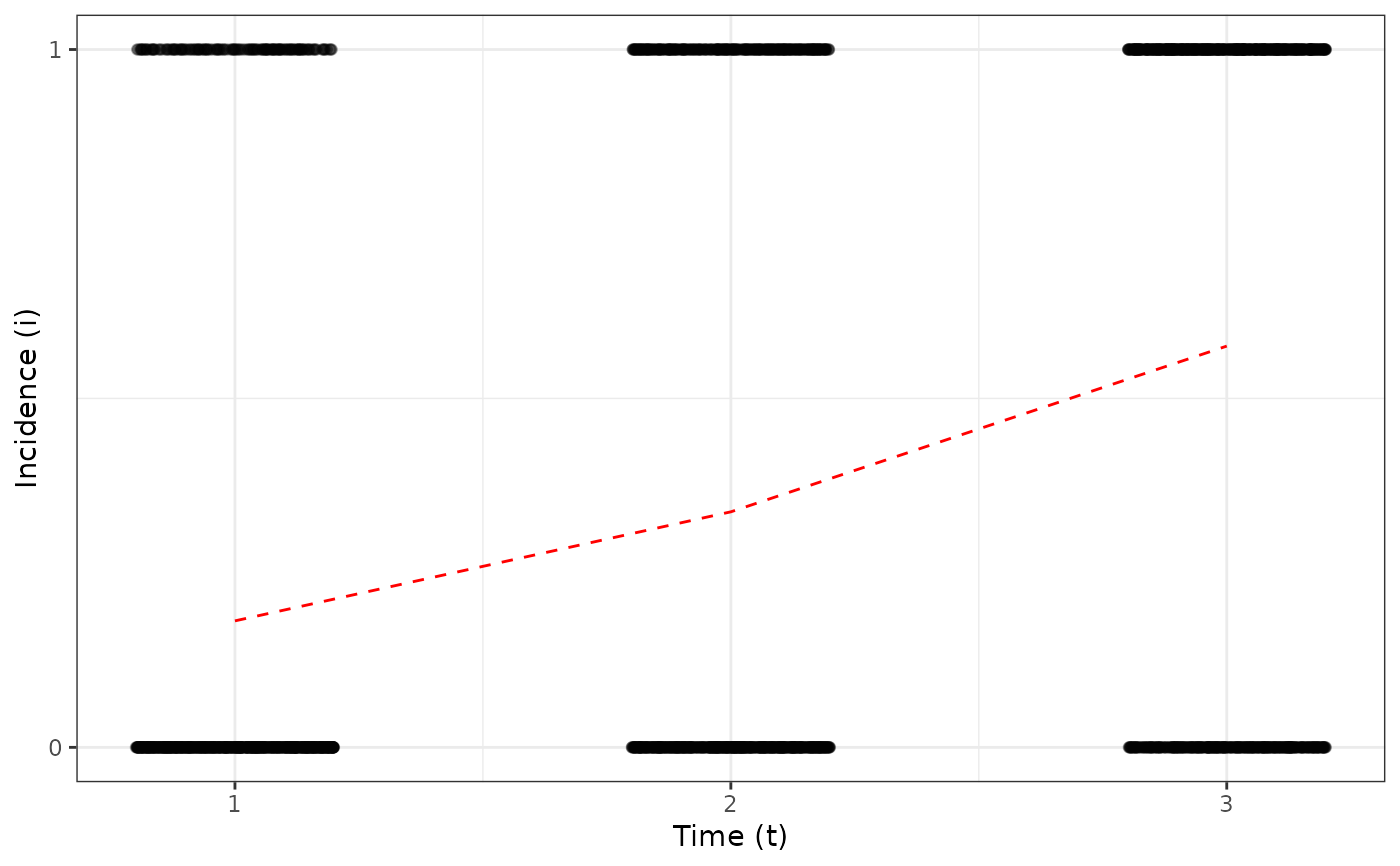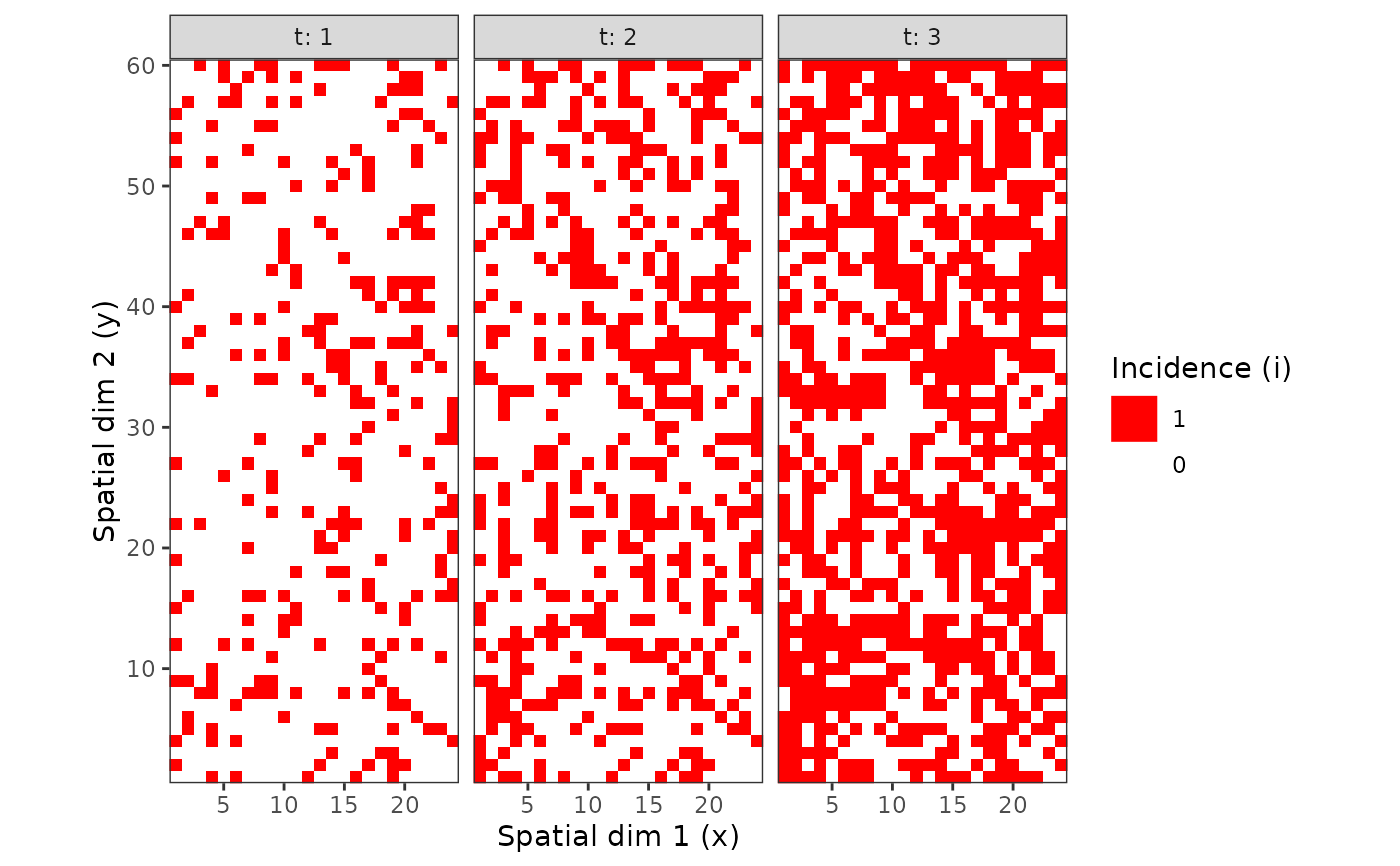# Different spatial size units:
my_incidence_clumped_1 <- clump(my_incidence, unit_size = c(x = 3, y = 3))
plot(my_incidence_clumped_1, type = "all")#> Warning: Computation failed in stat_summary():
#> Hmisc package required for this function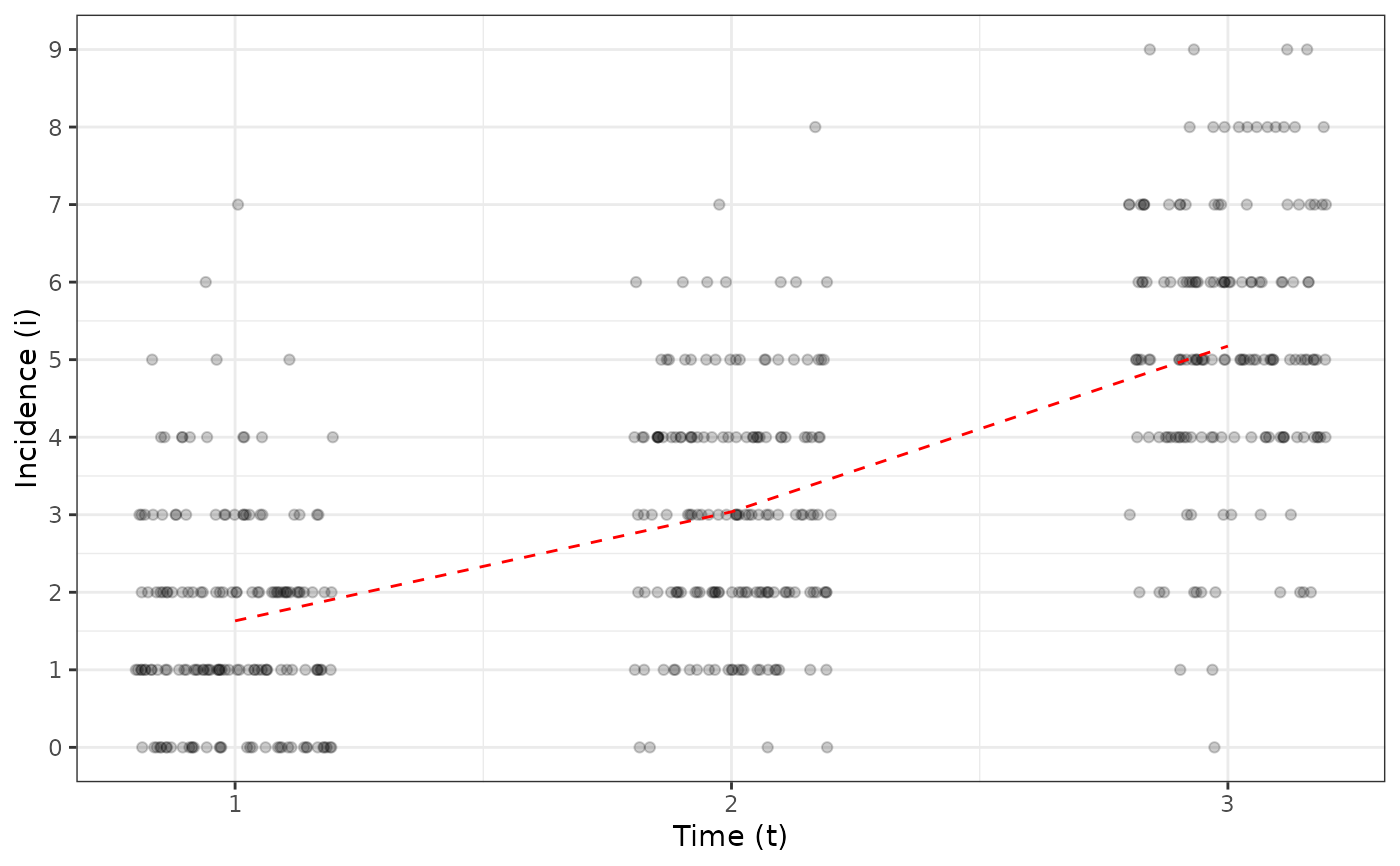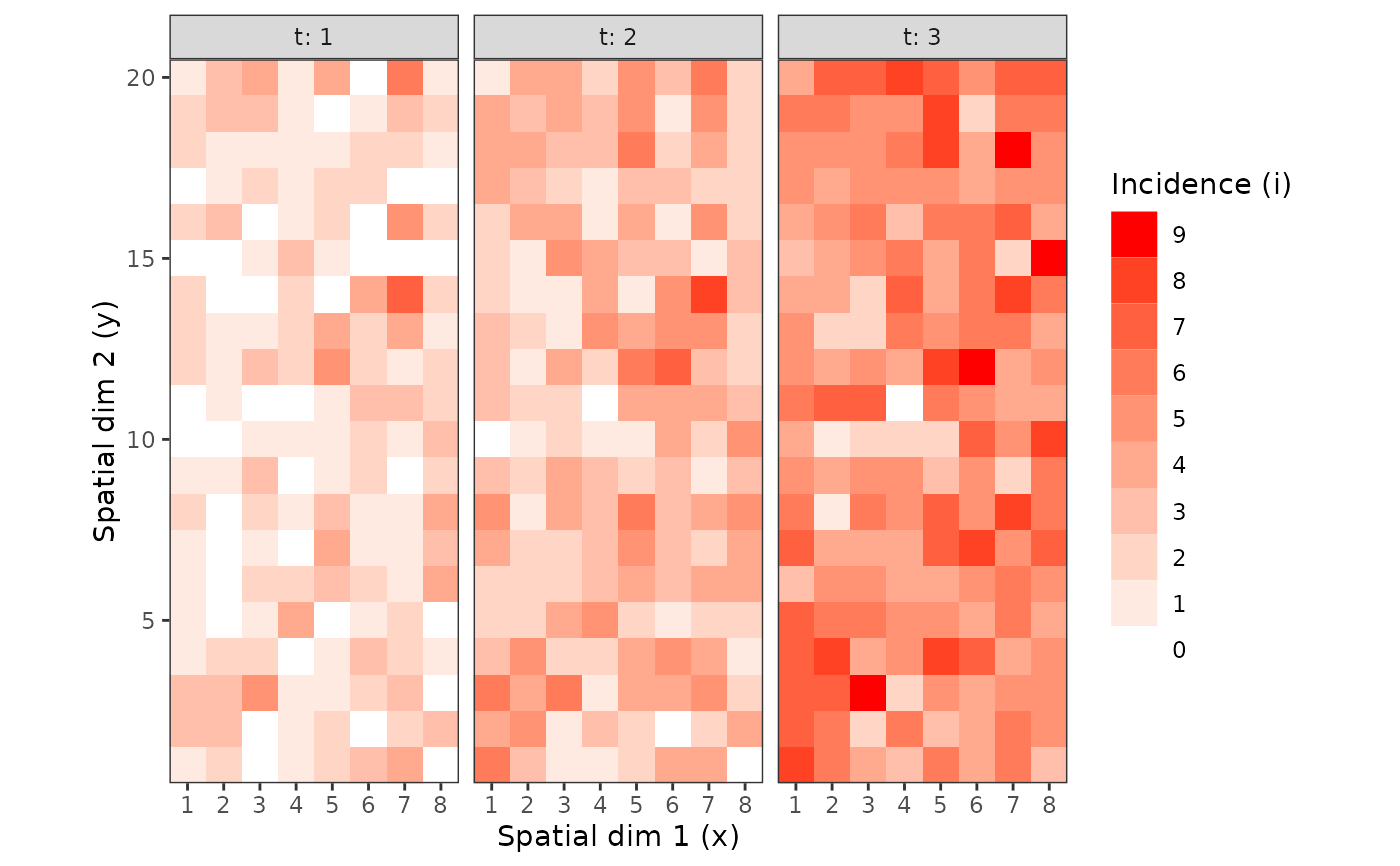my_incidence_clumped_2 <- clump(my_incidence, unit_size = c(x = 4, y = 5))
plot(my_incidence_clumped_2, type = "all")#> Warning: Computation failed in stat_summary():
#> Hmisc package required for this function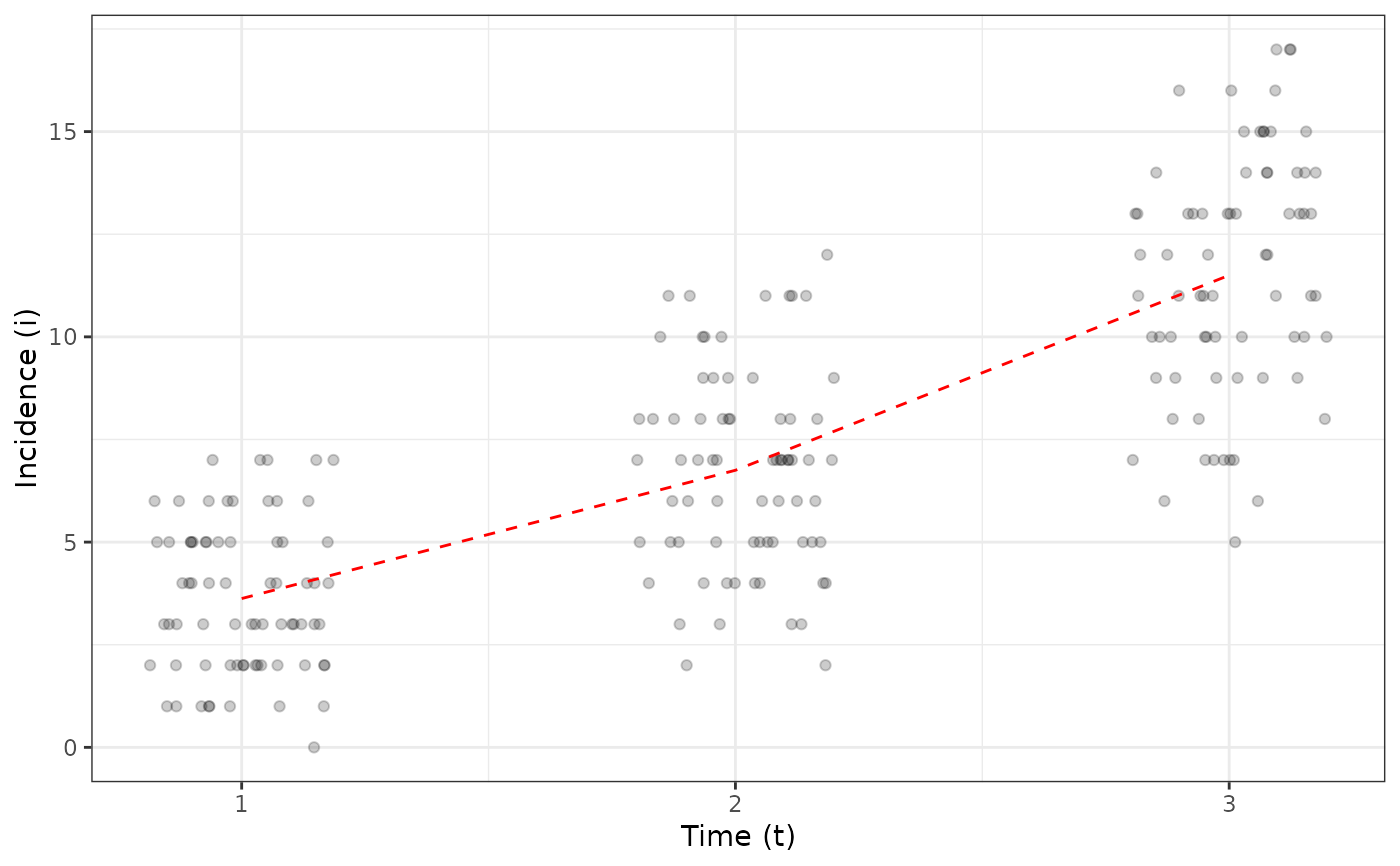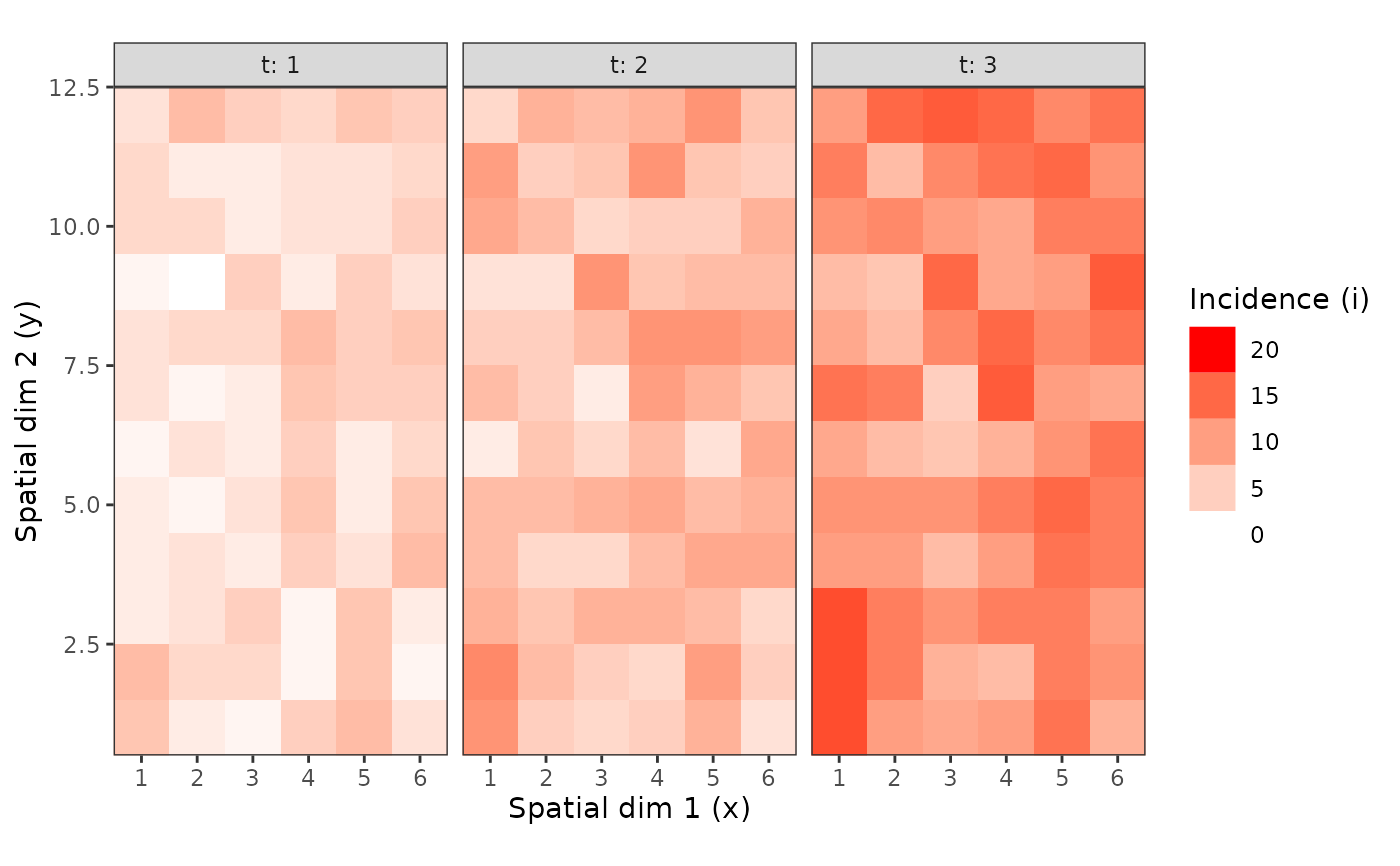# To get mean disease incidence for each plant over the 3 scoring dates:
my_incidence_clumped_3 <- clump(my_incidence, unit_size = c(t = 3), fun = mean)
plot(my_incidence_clumped_3)